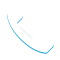### Introduction to IBM SPSS Modeler and Data Science (v18.1.1) – 0A008G CR

Details

Course Code: 0A008G

Brand: DS&BA – SPSS

Category: Analytics

Skill Level: Intermediate

Duration: 16.00H

Modality: CR

Audience

• Business analysts
• Data scientists
• Clients who are new to IBM SPSS Modeler or want to find out more about using it

Prerequisites

• It is recommended that you have an understanding of your business data

Short Summary

This course provides the fundamentals of using IBM SPSS Modeler and introduces the participant to data science.

Overview

This course provides the fundamentals of using IBM SPSS Modeler and introduces the participant to data science. The principles and practice of data science are illustrated using the CRISP-DM methodology. The course provides training in the basics of how to import, explore, and prepare data with IBM SPSS Modeler v18.1.1, and introduces the student to modeling.

Topic

1. Introduction to data science
• List two applications of data science
• Explain the stages in the CRISP-DM methodology
• Describe the skills needed for data science
2. Introduction to IBM SPSS Modeler
• Describe IBM SPSS Modeler’s user-interface
• Work with nodes and streams
• Generate nodes from output
• Use SuperNodes
• Execute streams
• Open and save streams
• Use Help
3. Introduction to data science using IBM SPSS Modeler
• Explain the basic framework of a data-science project
• Build a model
• Deploy a model
4. Collecting initial data
• Explain the concepts “data structure”, “of analysis”, “field storage” and “field measurement level”
• Import Microsoft Excel files
• Import IBM SPSS Statistics files
• Import text files
• Import from databases
• Export data to various formats
5. Understanding the data
• Audit the data
• Check for invalid values
• Take action for invalid values
• Define blanks
6. Setting the of analysis
• Remove duplicate records
• Aggregate records
• Expand a categorical field into a series of flag fields
• Transpose data
7. Integrating data
• Append records from multiple datasets
• Merge fields from multiple datasets
• Sample records
8. Deriving and reclassifying fields
• Use the Control Language for Expression Manipulation (CLEM)
• Derive new fields
• Reclassify field values
9. Identifying relationships
• Examine the relationship between two categorical fields
• Examine the relationship between a categorical field and a continuous field
• Examine the relationship between two continuous fields
10. Introduction to modeling
• List three types of models
• Use a supervised model
• Use a segmentation model

Objectives

• Introduction to data science
• Introduction to IBM SPSS Modeler
• Introduction to data science using IBM SPSS Modeler
• Collecting initial data
• Understanding the data
• Setting the of analysis
• Integrating data
• Deriving and reclassifying fields
• Identifying relationships
• Introduction to modeling

Tags :Call Now +27 72 266 2599